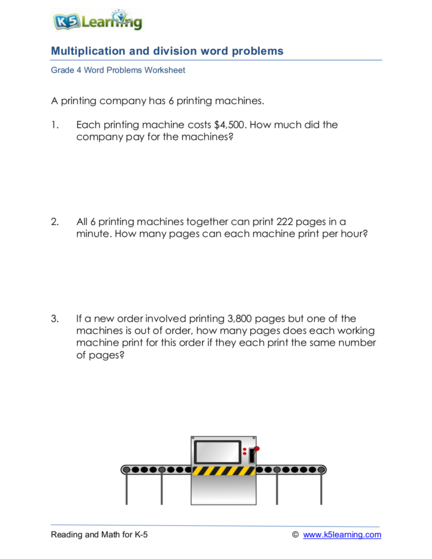# Multiplication and Division Word/Story ProblemsContributed by:Multiplication and division word/story problems. A word problem is a mathematical exercise where significant background information on the problem is presented in ordinary language rather than in mathematical notation.
1. Multiplication and division word problems
A printing company has 6 printing machines.
1. Each printing machine costs \$4,500. How much did the
company pay for the machines?
2. All 6 printing machines together can print 222 pages in a
minute. How many pages can each machine print per hour?
3. If a new order involved printing 3,800 pages but one of the
machines is out of order, how many pages does each working
machine print for this order if they each print the same number
of pages?
2. 4. There are 3 teams of maintenance staff. If each team take 8-
hour shifts and has 4 members, how many maintenance staff
are there?
5. There are 5 salespersons and 22 clients. If each client only deals
with one salesperson and the clients are divided as equally as
possible among the salespersons, how many salespersons are
responsible for 5 clients?
6. Write an equation using “x” and then solve the equation.
Three orders are to be done today. Each order involves printing
x pages. As a result, there are total of 9900 pages to be printed
today.
3. 1. 4,500 x 6 = 27,000
The company paid \$27,000 for the machines.
2. 222 ÷ 6 = 37
Each machine can print 37 pages per minute.
37 x 60 = 2,220
Each machine can print 2,220 pages per hour.
3. 3,800 ÷ 5 = 760
Each machine will print 760 pages.
4. 3 x 4 = 12
There are 12 maintenance staff.
5. 22 ÷ 5 = 4 remainder 2
2 salespersons are responsible for 5 clients.
6. 3x = 9,900
x = 3,300
Each order has 3,300 pages.